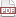### Tip 50: Optional and named arguments

09 January 12. [link]PDF version

level: comfortable with designated initializers
purpose: the awesomeness is self-evident

Part of a series of tips on POSIX and C. Start from the tip intro page, or get 21st Century C, the book based on this series.

I've already shown you how you can send a list of identical arguments to a function more cleanly via compound literal + designated initializer + variable-length macro. If you don't remember, go read Tip #26 right now.

A struct is in many ways, just like an array, but holding not-identical types, so it seems like we could apply the same routine. Let's go!

#include <stdio.h>

typedef struct{
double pressure, moles, temp;
} ideal_struct;

/** Find the volume (in cubic meters) via the ideal gas law: V =nRT/P
Inputs:
pressure in atmospheres (default 1)
moles of material (default 1)
temperature in Kelvins (default freezing = 273.15)
*/
#define ideal_pressure(...) ideal_pressure_base((ideal_struct){.pressure=1, .moles=1, .temp=273.15, __VA_ARGS__})

double ideal_pressure_base(ideal_struct in){
return 8.314 * in.moles*in.temp/in.pressure;
}

int main(){
printf("volume given defaults: %g\n", ideal_pressure() );
printf("volume given boiling temp: %g\n", ideal_pressure(.temp=373.15) );
printf("volume given two moles: %g\n", ideal_pressure(.moles=2) );
printf("volume given two boiling moles: %g\n", ideal_pressure(.moles=2, .temp=373.15) );
}


There are three parts to this: the throwaway struct, which the user will never directly name (but which still has to clutter up the global space if the function is going to be global); the macro that inserts its arguments into a struct, which then gets passed to the base function; and the base function.

Here's how the function call (don't tell the user, but it's actually a macro) on the last line will expand:

ideal_pressure_base((throwaway){.pressure=1, .moles=1, .temp=273.15, .moles=2, .temp=373.15})


The rule is that if an item is initialized multiple times, then the last initialization takes precedence. So .pressure is left at its default of one, while the other two inputs are set to the user-specified value.

I have an anonymous user on Stack Overflow to thank for pointing out the trick about setting defaults like this. I have to work out how to credit him/her.

All the other rules about designated initializers still hold, so if there were a natural order to the inputs, you wouldn't need to specify the names; in this case, ideal_pressure(2, 2) would do the math for 2 atmospheres and 2 moles.

The big problem I have with this function, as evidenced in the sample code, is that it's hard to work out where to put the documentation. C tools aren't written around having a macro that calls a function, so this can be awkward to do.

To do:
In this case, the throwaway struct may not be so throwaway, because it might make sense to run the formula in multiple directions:
pressure = 8.314 moles * temp/volume;
moles = pressure *volume /(8.314 temp);
temp = pressure *volume /(8.314 moles).
Rewrite the above struct to also have a volume element, and use the same struct to write the functions for these additional equations.

To do:
How could you write macros to make the production of the three parts needed for this trick easier to write? Though, regardless of how much typing it takes, having named, optional arguments is so supremely fabulous that it's worth the setup.